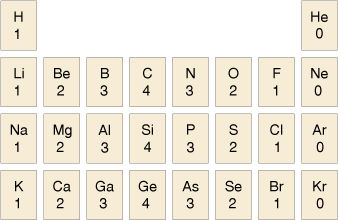Science, Maths & Technology

### Become an OU studentDiscovering chemistry

Start this free course now. Just create an account and sign in. Enrol and complete the course for a free statement of participation or digital badge if available.

# 3.2 Valency – the combining power of an atom

Elements have a tendency to form compounds with other elements in fixed ratios.

It is almost as though atoms have a set number of ‘hooks’ that can be used to link to other atoms. Of course, there are no actual hooks and the bonds between atoms are formed from their valence electrons.

Let’s look at a simple example.

Carbon forms many compounds with hydrogen and their formulas are consistent with carbon having four ‘hooks’.

• How many valence electrons does carbon have?

• The electronic configuration of the carbon atom is 1s 2 2s2 2p2. So, in the valence shell (in this case corresponding to the principal quantum number 2), there are four electrons. Here the number of valence electrons corresponds directly to the number of ‘hooks’.

Here the number of valence electrons corresponds directly to the number of hooks, the link is not always so simple, as you will see.

The simplest compound of carbon and hydrogen is the gas methane, CH4: a formula that would be obtained if it were assumed that carbon had four ‘hooks’ and hydrogen had one. Similarly the chlorine atom also has one ‘hook’, so the prediction for the formula of the compound from carbon and chlorine is CCl4.

This tendency for atoms to behave as though they have a specific number of ‘hooks’ is known as valency, and is sometimes referred to as the combining power of an atom (or a group of atoms).

The usual valency values of some elements are shown in Table 2. You should note the pattern here compares with the layout of the periodic table.Table 2 Valency of some more common elements